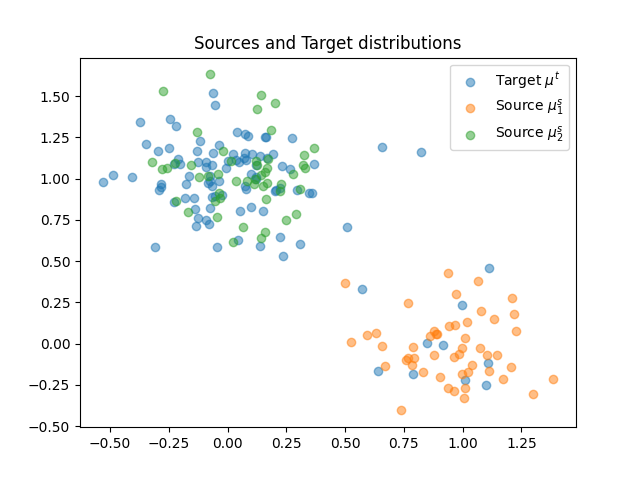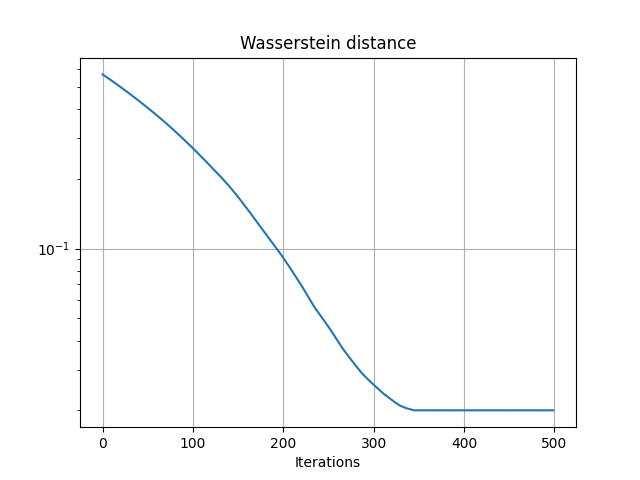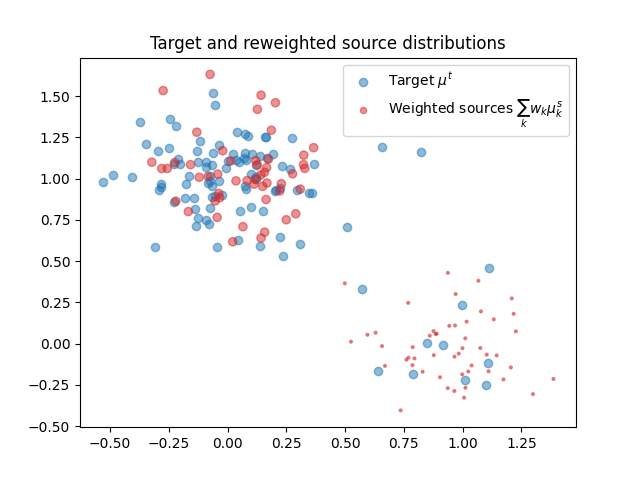# Wasserstein unmixing with PyTorch

In this example we estimate mixing parameters from distributions that minimize the Wasserstein distance. In other words we suppose that a target distribution $$\mu^t$$ can be expressed as a weighted sum of source distributions $$\mu^s_k$$ with the following model:

$\mu^t = \sum_{k=1}^K w_k\mu^s_k$

where $$\mathbf{w}$$ is a vector of size $$K$$ and belongs in the distribution simplex $$\Delta_K$$.

In order to estimate this weight vector we propose to optimize the Wasserstein distance between the model and the observed $$\mu^t$$ with respect to the vector. This leads to the following optimization problem:

$\min_{\mathbf{w}\in\Delta_K} \quad W \left(\mu^t,\sum_{k=1}^K w_k\mu^s_k\right)$

This minimization is done in this example with a simple projected gradient descent in PyTorch. We use the automatic backend of POT that allows us to compute the Wasserstein distance with ot.emd2 with differentiable losses.

# Author: Remi Flamary <remi.flamary@polytechnique.edu>
#

# sphinx_gallery_thumbnail_number = 2

import numpy as np
import matplotlib.pylab as pl
import ot
import torch


## Generate data

nt = 100
nt1 = 10  #

ns1 = 50
ns = 2 * ns1

rng = np.random.RandomState(2)

xt = rng.randn(nt, 2) * 0.2
xt[:nt1, 0] += 1
xt[nt1:, 1] += 1

xs1 = rng.randn(ns1, 2) * 0.2
xs1[:, 0] += 1
xs2 = rng.randn(ns1, 2) * 0.2
xs2[:, 1] += 1

xs = np.concatenate((xs1, xs2))

# Sample reweighting matrix H
H = np.zeros((ns, 2))
H[:ns1, 0] = 1 / ns1
H[ns1:, 1] = 1 / ns1
# each columns sums to 1 and has weights only for samples form the
# corresponding source distribution

M = ot.dist(xs, xt)


## Plot data

pl.figure(1)
pl.scatter(xt[:, 0], xt[:, 1], label='Target $\mu^t$', alpha=0.5)
pl.scatter(xs1[:, 0], xs1[:, 1], label='Source $\mu^s_1$', alpha=0.5)
pl.scatter(xs2[:, 0], xs2[:, 1], label='Source $\mu^s_2$', alpha=0.5)
pl.title('Sources and Target distributions')
pl.legend()Out:

<matplotlib.legend.Legend object at 0x7f5dbde4b190>


## Optimization of the model wrt the Wasserstein distance

# convert numpy arrays to torch tensors
H2 = torch.tensor(H)
M2 = torch.tensor(M)

# weights for the source distributions

# uniform weights for target
b = torch.tensor(ot.unif(nt))

lr = 2e-3  # learning rate
niter = 500  # number of iterations
losses = []  # loss along the iterations

# loss for the minimal Wasserstein estimator

def get_loss(w):
a = torch.mv(H2, w)  # distribution reweighting
return ot.emd2(a, b, M2)  # squared Wasserstein 2

for i in range(niter):

loss = get_loss(w)
losses.append(float(loss))

loss.backward()

w[:] = ot.utils.proj_simplex(w)  # projection on the simplex



## Estimated weights and convergence of the objective

we = w.detach().numpy()
print('Estimated mixture:', we)

pl.figure(2)
pl.semilogy(losses)
pl.grid()
pl.title('Wasserstein distance')
pl.xlabel("Iterations")Out:

Estimated mixture: [0.09980706 0.90019294]

Text(0.5, 23.52222222222222, 'Iterations')


## Ploting the reweighted source distribution

pl.figure(3)

# compute source weights
ws = H.dot(we)

pl.scatter(xt[:, 0], xt[:, 1], label='Target $\mu^t$', alpha=0.5)
pl.scatter(xs[:, 0], xs[:, 1], color='C3', s=ws * 20 * ns, label='Weighted sources $\sum_{k} w_k\mu^s_k$', alpha=0.5)
pl.title('Target and reweighted source distributions')
pl.legend()Out:

<matplotlib.legend.Legend object at 0x7f5da9c8adf0>


Total running time of the script: ( 0 minutes 1.454 seconds)

Gallery generated by Sphinx-Gallery